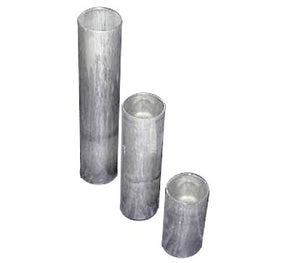# Round Candle Making Mold

• \$6.95

Some molds have flat tops and some have concave. If it is a concern please check prior to ordering. Round Aluminum molds are a candle making favorite.

Wash molds with warm soapy water before first use.  Use a soft cloth.  Dry well.

1.75" x 7" high. (Wish Candles) Aluminum flat 1 hole. 9 oz. wax weight

2.0" x 3.5" = 1 hole = 4.75 oz. wax weight

2.0" x 4.5" = 1 hole = 6 oz. wax weight

2.0" x 5.5" = 1 hole = 7.5 oz. wax weight

2.0" x 6.5" = 1 hole = 8.75oz. wax weight

2.5" x 3.5 " - 1 hole = 9 oz. wax weight

2.5" x 6.5" = 1 lb. wax weight

3.0" x 3.5" = 1 hole.  = 13 oz. wax weight

3.0" x 6.5" = 1 lb. 7 oz. wax weight

3.0" x 9.5" = 2 lbs. wax weight

3.5" x 3.5"  1 hole. 3.5" = 1 lb. wax weight

3.5" X 4.5" = 1 lb. 5 oz. wax weight

3.5" x 5.5" = 1 lb 10 oz. wax weight

4.0" x. 3.5" 1 hole. 3.5" = 1 lb. 6 oz. wax weight

4.0" x 6.5" = 2 lbs. 8 oz. wax weight

4.0" x 9.5" = 3 lbs. 9 oz. wax weight

5.0" x 5.5" diameter. 1 hole. 3 lbs. 3 oz. wax weight